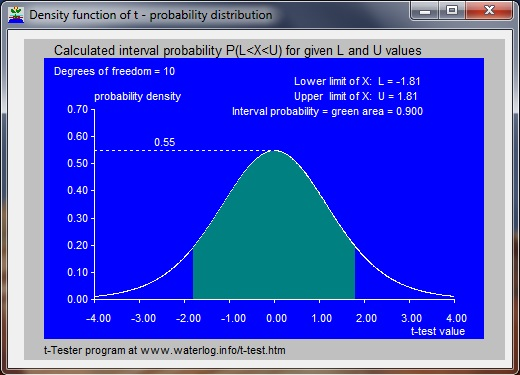# t-Tester, two-way calculator for Student's t-distribution with graphics   Entirely free download of software

 t-Tester is a calculator program using a model application (app) made with Delphi software for calculations with Student's t-distribution and performing the t-test. The difference between means (averages) of different samples from normally distributed random variables may, when divided by the standard deviation, follow a Student (t) probability distribution with N degrees of freedom. This property is used in the t-test by which it can be found whether the averages from different samples are significantly different or not. With Student's t-distribution one can calculate the cumulative probability (Pc) of any reference X-value (T, the t-test value), or, reversely, one can find the t-test value for any Pc. Pc(T) is the probability (P) that an X-value is less than the reference t-test value T : Pc(T) = P(X < T). The probability (P) that X occurs between a lower limit (L) and an upper limit (U) can be found from: P(L < X < U) = Pc(U) - Pc(L). Using L=-5, and Pc(L) = Pc(-5) = 0, one finds P(L < X < U) = Pc(U). The exceedance probability (Pe), being the probability that X is greater than t-test value equals 1 - Pc. Hence, when Pc is known, the Pe is also known. Screenprint of t-Tester, the two-way model calculator for the t- (probability) distribution:Experiences: For improvement, I am interested to learn about your experiences with t-Tester. For this, there is a contact form.

t-Tester
t-distribution calculator

Go to:

software
& models

articles
& manuals

reports
case studies

FAQ's
& papers

home
page

t-Tester software graphics in accordance to data in the above screenprint of the calculatorThe cumulative t-distribution of Student is used         in the T-tester model calculator to test the difference         between the means of data sets.The confidence interval of the difference of mean values of data series is shown in green color. This interval is used by T-tester model calculator in Student's t-test

 MATHEMATICS The t-Tester software model uses a numerical solution of the cumulative t- distribution function and from that it derives the t- probability density by numerical differentiation.   Numerical approximation of Student's t- (probability) distribution. Using:         T = reference value for the X-variable following the t-distribution, Po = intermediate probability variable,         Pc(T) = cumulative probability of T, and Pc(T) = P(X < T) , the following equations hold: N (degrees of freedom) even :         Po = sin(z) [1 + cos2(z) / 2 + 3 cos4(z) / 8 + 15 cos6(z) / 48 + 105 cos8(z) / 384 + . . . ] N (degrees of freedom) uneven (odd) and >1 :         Po = 2Z/pi + (2/pi)cos(z).sin(Z) [1 + 2 cos2(z) / 3 + 8 cos4(z) / 15 + 48 cos6(z) / 105 + . . . ] N (degrees of freedom) = 1 :         Po = 2z / pi where :         z = arctan(T / sqrt(N) , and pi = 22 / 7 Now :         when T is positive: Pc(T) = Po + (1-Po) / 2         when T is negative: Pc(T) = (1-Po) / 2 The number of terms between the parentheses [ ] to be used is N / 2 when N is even and (N-1) / 2 when N is uneven (odd).The flowers are here to make     the mathematics     less boring.